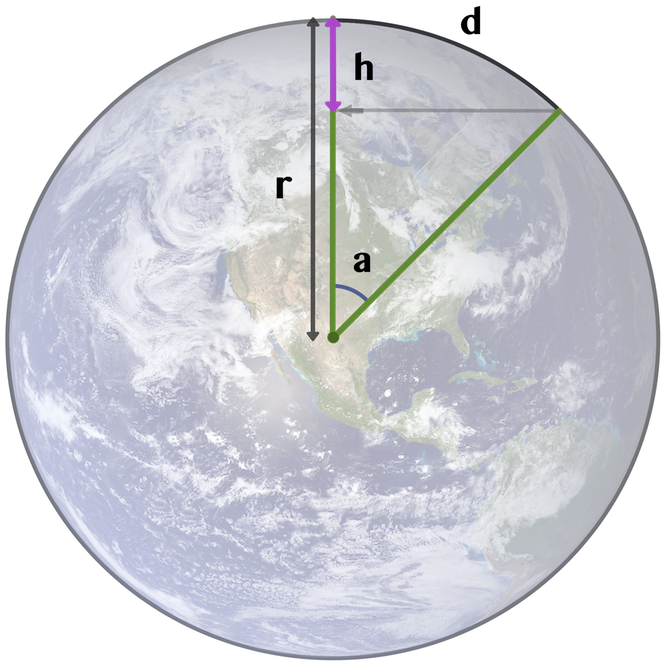# Earth Curvature Calculator

Accurately calculate the curvature you are supposed to see on the ball Earth.

Distance Curvature
1 km 0.00008 km = 0.08 meters
2 km 0.00031 km = 0.31 meters
5 km 0.00196 km = 1.96 meters
10 km 0.00785 km = 7.85 meters
20 km 0.03139 km = 31.39 meters
50 km 0.19620 km = 196.20 meters
100 km 0.78479 km = 784.79 meters
200 km 3.13897 km = 3138.97 meters
500 km 19.6101 km = 19610.09 meters
1000 km 78.3196 km = 78319.62 meters

## Explanation:

The Earth's radius `(r)` is 6371 km or 3959 miles, based on numbers from Wikipedia,
which gives a circumference `(c)`of `c = 2 * π * r = 40 030 km`

We wish to find the height `(h)` which is the drop in curvature over the distance `(d)`

Using the circumference we find that 1 kilometer has the angle
`360° / 40 030 km = 0.009°`. The angle `(a)` is then `a = 0.009° * distance (d)`

The derived formula `h = r * (1 - cos a)` is accurate for any distance `(d)`